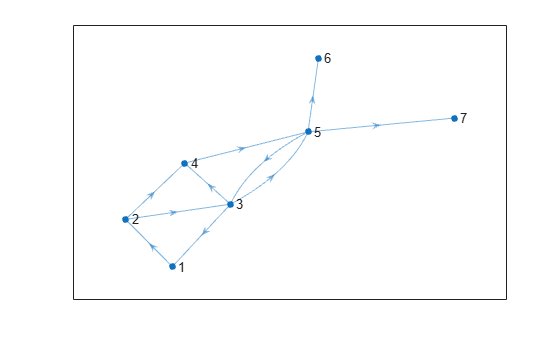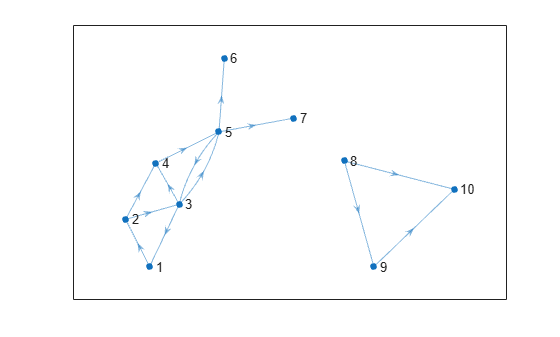conncomp

Connected graph components

Description

example

bins = conncomp(G) returns the connected components of graph G as bins. The bin numbers indicate which component each node in the graph belongs to.

• If G is an undirected graph, then two nodes belong to the same component if there is a path connecting them.

• If G is a directed graph, then two nodes belong to the same strong component only if there is a path connecting them in both directions.

example

bins = conncomp(G,Name,Value) uses additional options specified by one or more Name-Value pair arguments. For example, conncomp(G,'OutputForm','cell') returns a cell array to describe the connected components.

[bins,binsizes] = conncomp(___) also returns the size of the connected components. binsizes(i) gives the number of nodes in component i.

Examples

collapse all

Create and plot an undirected graph with three connected components. Use conncomp to determine which component each node belongs to.

G = graph([1 1 4],[2 3 5],[1 1 1],6);
plot(G)bins = conncomp(G)
bins = 1×6

1     1     1     2     2     3

Create and plot a directed graph, and then compute the strongly connected components and weakly connected components. Weakly connected components ignore the direction of connecting edges.

s = [1 2 2 3 3 3 4 5 5 5 8 8];
t = [2 3 4 1 4 5 5 3 6 7 9 10];
G = digraph(s,t);
plot(G,'Layout','layered')str_bins = conncomp(G)
str_bins = 1×10

4     4     4     4     4     6     5     1     3     2

weak_bins = conncomp(G,'Type','weak')
weak_bins = 1×10

1     1     1     1     1     1     1     2     2     2

Use the second output of conncomp to extract the largest component of a graph or to remove components below a certain size.

Create and plot a directed graph. The graph has one large component, one small component, and several components that contain only a single node.

s = [1 2 2 3 3 3 4 5 5 5 8 8 9];
t = [2 3 4 1 4 5 5 3 6 7 9 10 10];
G = digraph(s,t,[],20);
plot(G,'Layout','layered')Calculate the weakly connected components and specify two outputs to conncomp to get the size of each component.

[bin,binsize] = conncomp(G,'Type','weak')
bin = 1×20

1     1     1     1     1     1     1     2     2     2     3     4     5     6     7     8     9    10    11    12

binsize = 1×12

7     3     1     1     1     1     1     1     1     1     1     1

Use binsize to extract the largest component from the graph. idx is a logical index indicating whether each node belongs to the largest component. The subgraph function extracts the nodes selected by idx from G.

idx = binsize(bin) == max(binsize);
SG = subgraph(G, idx);
plot(SG)A similar use of binsizes is to filter out components based on size. The procedure is similar to extracting the largest component, however in this case each node can belong to any component that meets the size requirement.

Filter out any components in G that have fewer than 3 nodes. idx is a logical index indicating whether each node belongs to a component with 3 or more nodes.

idx = binsize(bin) >= 3;
SG = subgraph(G, idx);
plot(SG)Input Arguments

collapse all

Input graph, specified as either a graph or digraph object. Use graph to create an undirected graph or digraph to create a directed graph.

Example: G = graph(1,2)

Example: G = digraph([1 2],[2 3])

Name-Value Arguments

Specify optional comma-separated pairs of Name,Value arguments. Name is the argument name and Value is the corresponding value. Name must appear inside quotes. You can specify several name and value pair arguments in any order as Name1,Value1,...,NameN,ValueN.

Example: bins = conncomp(G,'OutputForm','cell')

Type of output, specified as the comma-separated pair consisting of 'OutputForm' and either 'vector' or 'cell'.

OptionOutput
'vector' (default)bins is a numeric vector indicating which connected component each node belongs to.
'cell'bins is a cell array, and bins{j} contains the node IDs for all nodes that belong to component j.

Note

The 'Type' option is supported only for directed graphs created using digraph.

Type of connected components, specified as the comma-separated pair consisting of 'Type' and either 'strong' (default) or 'weak'.

OptionResult
'strong' (default)Two nodes belong to the same connected component only if there is a path connecting them in both directions.
'weak'Two nodes belong to the same connected component if there is a path connecting them, ignoring edge directions.

Example: bins = conncomp(G,'Type','weak') computes the weakly connected components of directed graph G.

Output Arguments

collapse all

Connected components, returned as a vector or cell array. The bin numbers assign each node in the graph to a connected component:

• If OutputForm is 'vector' (default), then bins is a numeric vector indicating which connected component (bin) each node belongs to.

• If OutputForm is 'cell', then bins is a cell array, with bins{j} containing the node IDs for all nodes that belong to component j.

Size of each connected component, returned as a vector. binsizes(i) gives the number of elements in component i. The length of binsizes is equal to the number of connected components, max(bins).

collapse all

Weakly Connected Components

Two nodes belong to the same weakly connected component if there is a path connecting them (ignoring edge direction). There are no edges between two weakly connected components.

The concepts of strong and weak components apply only to directed graphs, as they are equivalent for undirected graphs.

Strongly Connected Components

Two nodes belong to the same strongly connected component if there are paths connecting them in both directions. There can be edges between two strongly connected components, but these connecting edges are never part of a cycle.

The bin numbers of strongly connected components are such that any edge connecting two components points from the component of smaller bin number to the component with a larger bin number.

The concepts of strong and weak components apply only to directed graphs, as they are equivalent for undirected graphs.